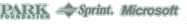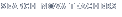Back to Teachers HomeInfinite SecretsClassroom ActivityObjective
To duplicate the method Archimedes used to estimate the value of pi.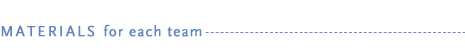• copy of the "Archimedes' Recipe for Pi" student handout (PDF or HTML)
• paper
• pencil
• compass
• ruler
• calculator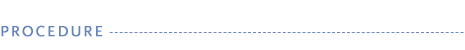1. Tell students that they will be exploring Archimedes' method for estimating the value of pi, a mathematical constant that is the ratio of a circle's circumference (the distance around a circle) to its diameter (the distance across a circle through its center). The Greek symbol for pi is pi.

2. Organize students into groups. Provide copies of the "Archimedes' Recipe for Pi" student handout and other materials to each group.

3. Define some terms for students: perimeter, circumference, radius, diameter, and area. (See Activity Answer for more information.)

4. Demonstrate how to draw polygons that are inscribed in a circle and circumscribed around a circle:

• Draw a circle on the blackboard.

• Use a ruler to draw four lines that divide the circle into eight equal parts, extending the lines beyond the boundary of the circle.

• Connect the points where the lines meet the inside of the circle to create an octagon.

• Connect the lines around the outside of the circle to create another octagon that just touches the edge of the circle.

5. Point out that the perimeters of the polygons give approximate values for the circumference of the circle. Students can divide each polygon perimeter by the diameter of the circle to find an approximate value for pi. (If necessary, remind students that to find the perimeter of a regular polygon they can measure one side of a polygon and multiply that length by the number of sides.)

6. Have students complete the data table and find the approximate values of pi for each of the three suggested sets of polygons (square, octagon, and hexadecagon) described on the student handout.

7. When students have finished the activity, discuss their results using the questions on the student handout.

8. As an extension, have students develop fact sheets about pi.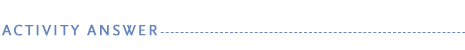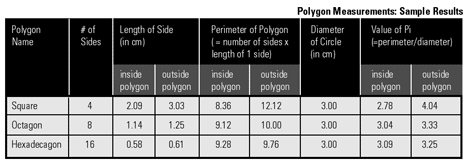If students need guidance, you may want to clarify the following concepts:

perimeter: the distance around a polygon; the perimeter is determined by the sum of the lengths of the sides of the polygon.
circumference: the distance around a circle; the circumference is the perimeter of a circle.
radius: any segment from the center of a circle to its edge.
diameter: any segment from one side of a circle to the other through the circle's center; the diameter is the same length as two radii.
area: the amount of space included within a polygon or circle.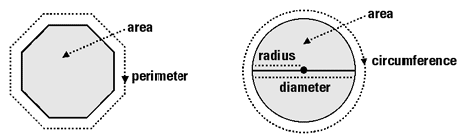For any circle, if you divide the circle's circumference by the diameter you will always get the same number, pi. Pi represents the ratio of the circumference of a circle to its diameter. Pi can be used to find the circumference and the area of a circle, if you know what the radius (r) of the circle is. The equations for determining those values are:

Circumference = 2 x π x r
Area = π x r x r

The concept of pi has fascinated mathematicians for more than 4,000 years, ever since people first noticed that the ratio of circumference to diameter was the same for all circles, regardless of the circle's size. Although earlier estimates of the value of pi exist, Archimedes seems to have carried out the first theoretical calculation of the constant. His approach consisted of inscribing and circumscribing regular, many-sided polygons in and around the circle, and computing the perimeters of these polygons. This provided him with the approximation 223/71 < π < 22/7, or 3.1408 < π < 3.1428. (The actual value of pi to four decimal places is 3.1415.) Pi is an infinite decimal; its value is currently known to more than 1 trillion decimal places.

Archimedes did not have access to the modern-day tools of algebra, trigonometry, or even decimal notation. Instead, he performed his calculations using purely geometrical methods. Thus, constructing and calculating the values of the perimeters of 96-sided polygons, and then using these values to estimate pi, was by no means a trivial task.

Students' answers should reflect that Archimedes' method gives an approximate range of values for pi, with the value from the inside polygon providing the lower boundary and the value from the outside polygon providing the upper boundary. In addition, students should discover that the more sides a polygon has, the better approximation of pi it provides. This is because a polygon with many sides gives a better approximation of the circumference of the circle than one with fewer sides.

Students may wonder why it was so difficult to measure the circumference of a circle. The reason is that at the time Archimedes lived, there was no way to accurately measure curved lines—only straight lines could be measured. This is why Archimedes had to devise a way to approximate a circle's circumference.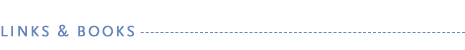Book

Stein, Sherman. Archimedes: What Did He Do Besides Cry Eureka? Washington, DC: The Mathematical Association of America, 1999.
Describes the life of Archimedes, the discovery of his manuscript in 1906, and his methods for figuring out many of the concepts he developed.

Web Sites

www.mcs.drexel.edu/~crorres/Archimedes/contents.html
Includes information on Archimedes' life and work as well as illustrations of his inventions.

Find more resources in Links and Books online at www.pbs.org/nova/archimedes/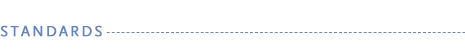The "Archimedes' Recipe for Pi" activity aligns with the following National Science Education Standards and Standards for School Mathematics.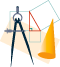Mathematics Standard 12: Geometry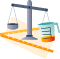Mathematics Standard 13: MeasurementMathematics Standard 12: GeometryMathematics Standard 13: Measurement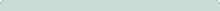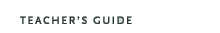Infinite SecretsOriginal broadcast:September 30, 2003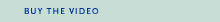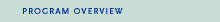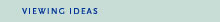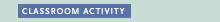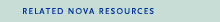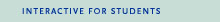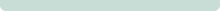Major funding for NOVA is provided by the Park Foundation, Sprint, and Microsoft